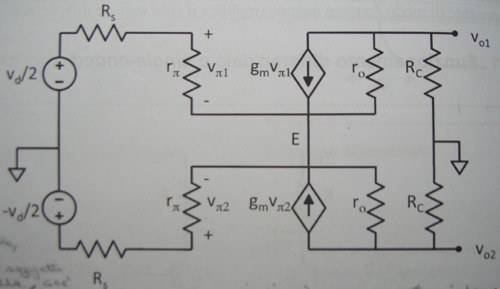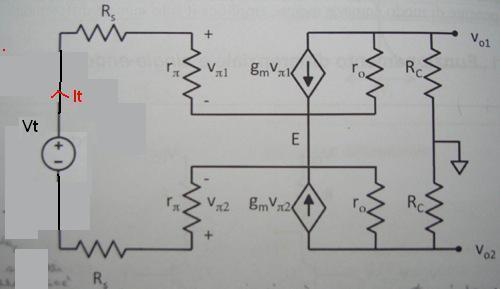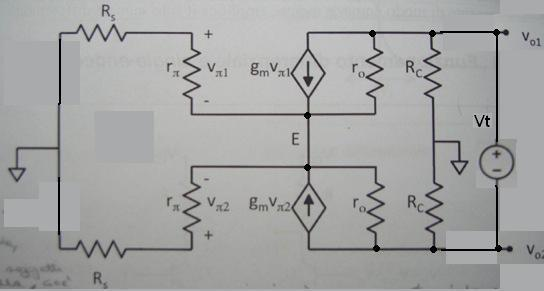# BJT differential amplifier, small signal analysis

eliotsbowe
Hello, I'm having some issues with small-signal analysis of BJT differential amplifiers.

Let's say I have this BJT differential pair and suppose that Q1 and Q2 perfectly match: http://i50.tinypic.com/14ahdgn.jpg

Suppose a small differential input is applied and consider the small–signal equivalent circuit:Having two opposite-phase inputs with the same amplitude ($\frac{v_d}{2} , - \frac{v_d}{2}$) makes the potential of the emitter E stuck at its initial value.

My book says that:
- If the potential of E is stuck at its value, then E can be considered as a ground point for the small-signal circuit;
- If E is grounded, then every point the two circuits have in common is grounded; this means that the two circuits become totally independent one from each other and I can solve the small-signal circuit of Q1 and Q2 separately;

Can anyone please explain me the reason for these two statements?

One last question: both small-signal circuits of Q1 and Q2 have an input impedance $r_\pi$ and an output impedance Rc || $r_o$.
What's the input/output impedance of the whole differential pair?

eliotsbowe
Thanks. I managed to solve the first two questions and I found something I was looking for in the pdf you've linked.

Now there's one pending question: what's the input/output impedance of the differential couple?

I mean: if I connect some circuit component to the two inputs of the differential amplifier, what impedance is the component going to "see"?

yungman
Don't quote me on this as it's been a long time. I believe is $2βr_e=2\frac β {g_m}\;$. The emitter of one transistor only see the $r_e\;$ of the other, assuming the tail resistor is much higher resistance.

eliotsbowe
Well, thanks for trying, but I'm interested in the procedure to find the two impedances!

yungman
I am pretty sure it is correct, input impedance of diff pair is not low. It become high impedance only when you add negative feedback and move the other side to follow the input.

Think about a normal diff pair in an opamp, the tail current say is 10uA. So it is 5uA each.

$$r_e=1/gm= \frac {V_T}{I_c}≈\frac {25mV}{5\mu A}=5000,\;\; β=100\;\Rightarrow 2β r_e= 1MΩ$$

That is in the ball part of the input impedance of some opamp when it is open loop. The impedance only get higher due to negative feedback.

Last edited:
Ratch
eliotsbowe,

Now there's one pending question: what's the input/output impedance of the differential couple?

I mean: if I connect some circuit component to the two inputs of the differential amplifier, what impedance is the component going to "see"?

You can tell just from inspection of the schemat what the impedances will be. Assuming ro is very high, the input circuit goes through the two r_pi resistors, so the impedance will be 2*r_pi. The output impedance is 2*Rc by inspection if ro is very large. Otherwise I would think it would be 2*Rc||ro/(β+1), because the output impedance of a single CE transistor diminishes by ro/(β+1)

Ratch

Jony130
I disagree with output impedance of a single CE amplifier is not equal ro/(β+1).
As for differential output impedance Rout = (2Rc*ro)/(Rc + ro)

eliotsbowe
Okay, I think I got it.
Just one thing: to compute the input impedance of a circuit, I usually disconnect the signal generator and its own resistance and replace it with a test generator, let's say a voltage generator Vx. The input impedance is defined as Vx / Ix , where Ix is the current flowing through the generator.

If I want to apply this method to the differential pair, I guess I should disconnect both $\frac{v_d}{2}$ and $-\frac{v_d}{2}$ (and the two $R_s$ resistors) and replace them with a test generator connected between the positive terminals of the two $r_\pi$ resistors.
Is this right?

Jony130
Yes, you use this circuit to find RinAnd this one to find RoutAnd from this diagram when we assume full symmetry. From the inspection we can see that
Rout = Vt/It = 2Rc||2ro

#### Attachments

Last edited:
eliotsbowe
Ok, thanks!

Ratch
Jony130,

Sorry I did not get back sooner.

I disagree with output impedance of a single CE amplifier is not equal ro/(β+1).
As for differential output impedance Rout = (2Rc*ro)/(Rc + ro)

Yes, you are correct. The output impedance of a CE is ro/(β+1) only if it is driven by a current source. Otherwise, in this voltage driven case, it is ro as you pointed out.

Ratch